Author: Sophia Tutorial
##### Description:

Calculate a company's total leverage.

(more)### Developing Effective Teams

*No strings attached. This college course is 100% free and is worth 1 semester credit.

37 Sophia partners guarantee credit transfer.

299 Institutions have accepted or given pre-approval for credit transfer.

* The American Council on Education's College Credit Recommendation Service (ACE Credit®) has evaluated and recommended college credit for 32 of Sophia’s online courses. Many different colleges and universities consider ACE CREDIT recommendations in determining the applicability to their course and degree programs.

Tutorial

what's covered
In this lesson, you will learn about a company's total leverage. Specifically, this lesson will cover:
1. Defining Financial Leverage
2. Impacts of Financial Leverage
1. Impact on Return on Equity
2. Leverage, Risk, and Misconceptions
3. Combining Operating Leverage and Financial Leverage

## 1. Defining Financial Leverage

At its simplest, leverage is a tactic geared at multiplying gains and losses. Leveraging existing assets to get exponentially more return can be a risk-intensive process and represents a significant aspect of financial strategy and capital structure. Achieving leverage can enable significant competitive advantages despite the risk and can also accelerate the speed of revenue acquisition exponentially.

The standard way to accomplish leverage is through borrowing, via debt and equity, to invest at a much higher scale than one’s current assets would allow. In order to borrow substantial amounts of capital, firms must pursue a variety of financial sourcing and be able to back up their debts with valuable assets, or collateral. Even with a great deal of collateral, borrowing big means risking big. Interest rates ensure that the strategic discussions around expanding leverage take into account the risk and return trade-offs.

The standard definition of financial leverage is a tactic to multiply gains and losses, calculated by a debt-to-equity ratio with the following formula:

formula

Financial Leverage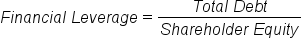In short, the ratio between debt and equity is a strong sign of leverage. As you may already know, equity is ownership of the organization and pays out fairly significant dividends. Debt is often lower cost access to capital, as debt is paid out before equity in the event of a bankruptcy (thus debt is an intrinsically lower risk for the investor).

The debt-to-equity ratio also plays a role in the working average cost of capital (WACC), as the overall interest on financing represents the break-even point that must be obtained to achieve profitability in a given venture. WACC is essentially the overall average interest an organization owes on the capital it has borrowed for leverage.

### IN CONTEXT

Suppose equity represents 60% of borrowed capital and debt is 40%. This results in a financial leverage calculation of 40/60, or 0.6667.

If the organization owes 10% on all equity and 5% on all debt, what is the weighted average cost of capital?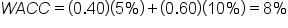That means that the weighted average cost of capital is 8%. For every \$10,000 borrowed, this organization will owe \$800 in interest. Profit must be higher than 8% on the project to offset the cost of interest and justify this leverage.

Leverage is exponentially riskier the more it is utilized. A useful way to view leverage is the overall existing assets of an organization compared to the amount of money they owe.

EXAMPLE

Suppose you own a company with an overall net worth of \$1 million. If you were to be leveraged at a total of 1.5 times (i.e., financed at \$1.5 million), this would put you at some risk – arguably, a reasonable amount.

EXAMPLE

Before Lehman Brothers went bankrupt, they were leveraged at over 30 times; \$691 billion in financial leverage compared to \$22 billion in assets. A mistake of this scale, on both the lenders and the Lehman Brothers, threatened to topple the global economy itself.

big idea
It is important to keep responsibility, accountability, and risk in mind when considering leverage options.

term to know

Financial Leverage
A tactic to multiply gains and losses, calculated by a debt-to-equity ratio.

## 2. Impacts of Financial Leverage

Taking on debt, as an individual or a company, will always bring about a heightened level of risk due to the fact that income must be used to pay back the debt even if earnings or cash flows go down. From a company’s perspective, the use of financial leverage can positively – or sometimes negatively – impact its return on equity as a consequence of the increased level of risk.

2a. Impact on Return on Equity
Return on equity is the rate of return on the shareholders’ equity of a company’s common stock owners. It measures a firm’s efficiency at generating profits from every unit of shareholders’ equity. Return on equity shows how well a company uses investment funds to generate earnings growth. It can be calculated using the following equation:

formula

Return on Equity (ROE)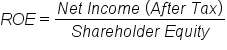At an ideal level of financial leverage, a company’s return on equity increases because the use of leverage increases stock volatility, which increases its level of risk, which in turn increases returns.

However, if a company is financially over-leveraged, a decrease in return on equity could occur. Financial over-leveraging means incurring a huge debt by borrowing funds at a lower rate of interest and using the excess funds in high risk investments. If the risk of the investment outweighs the expected return, the value of a company’s equity could decrease as stockholders believe it to be too risky.

2b. Leverage, Risk, and Misconceptions
The most obvious risk of leverage is that it multiplies losses. Due to financial leverage’s effect on solvency, a company that borrows too much money might face bankruptcy during a business downturn, while a less-leveraged company may avoid bankruptcy due to higher liquidity. There is a popular prejudice against leverage rooted in the observation of people who borrow a lot of money for personal consumption – for instance, heavy use of credit cards. However, in finance, the general practice is to borrow money to buy an asset with a higher return than the interest on the debt. Instead of spending money it doesn’t have, a company actually creates value. On the other hand, when debt is taken on for personal use, there is no value being created (i.e., no leveraging).

There is also a misconception that companies enter a higher level of financial leverage out of desperation, referred to as involuntary leverage. While involuntary leverage is certainly not a good thing, it is typically caused by eroding equity value as opposed to the addition of more debt. Therefore, it is typically a symptom of the problem, not the cause.

When evaluating the riskiness of leverage it is also important to factor in the value of the company itself and its activities. If a company borrows money to modernize, add to its product line, or expand internationally, the additional diversification will likely offset the additional risk from leverage. The upshot is, if value is expected to be added from the use of financial leverage, the added risk should not have a negative effect on a company or its investments.

## 3. Combining Operating Leverage and Financial Leverage

Operating and financial leverage can be combined into an overall measure called “total leverage.” Total leverage can be used to measure the total risk of a company and can be defined as the percentage change in stockholder earnings for a given change in sales. In other words, total leverage measures the sensitivity of earnings to changes in the level of a company’s sales.

Total leverage can be determined by a couple of different methods. If the percentage change in earnings and the percentage change in sales are both known, a company can simply divide the percentage change in earnings by the percentage change in sales. Earnings can be measured in terms of EBIT, earnings before interest and taxes, or EPS, earnings per share. While EBIT can be determined by referencing a company’s income statement, we can determine earnings per share by dividing the company’s net income by its average price of common shares.

Another way to determine total leverage is by multiplying the Degree of Operating Leverage and the Degree of Financial Leverage.

Total Leverage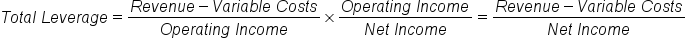In the fully derived formula, the numerator is revenue minus variable costs, also known as the total contribution margin. This can be calculated by subtracting unit variable costs from unit revenue, and then multiplying by the number of units sold. The denominator is the net income, which is equivalent to subtracting fixed costs and interest expense from the total contribution margin. Using this, we can express the total leverage with the following formula:

formula

Total Leverage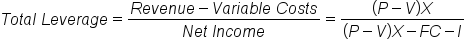In this formula:
• P is the unit revenue
• V is the unit variable costs
• X is the units sold
• FC is the fixed costs
• I is the interest expense
hint
Companies usually choose one form of leverage over the other when analyzing potential investments. One that utilizes both forms of leverage undertakes a very high level of risk.

summary
In this lesson, you learned that financial leverage is defined as a company’s ratio between debt and equity. The impact of leverage is such that risk increases as leverage increases. Leverage can also have an impact on return on equity by increasing it if a company is ideally leveraged, or decreasing it if the company is over-leveraged. There are some risks associated with financial leverage, especially if a company borrows too heavily, but there is also a misconception that debt is always bad. This is not true when debt is managed appropriately, because it helps companies to create value.

You also learned that combining operating leverage and financial leverage gives an overall measure of risk to a company known as “total leverage.” It is a measure of the sensitivity of shareholder earnings to changes in the level of a company’s sales.

Best of luck in your learning!

Source: THIS CONTENT HAS BEEN ADAPTED FROM LUMEN LEARNING'S “Thinking About Financial Leverage” TUTORIAL.

Terms to Know
Financial Leverage

A tactic to multiply gains and losses, calculated by a debt-to-equity ratio.

Formulas to Know
Financial LeverageReturn on Equity (ROE)Total LeverageRating